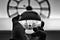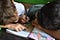# Understanding GANs — Deriving the Adversarial loss from scratch

Generative adversarial networks or GANs for short are an unsupervised learning task where the generator model learns to discover patterns in the input data in such a way that the model can be used to generate new samples of the training data.

The main idea of GAN is adversarial training, where two neural networks fight against each other and improve themselves to fight better.

# Confidence Intervals — A Gentle Introduction

In statistics, Confidence Interval is one of the most fundamental and important concept that is extensively used in Data Analysis and Machine Learning. In this blog, we will first understand why Confidence Intervals (CI) are important and then we will get into the theory behind it.

First and foremost,

## Why understanding Confidence Intervals (CI) is important?

Assume, you want to calculate the average salary of all the working professionals in the world and you want to be as accurate as possible. How will you do that?

Case 1: One easy way of solving this problem is to take reasonable amount of randomly chosen samples of people salary…

# Logistic Regression — A Geometric PerspectivePhoto by Jossuha Théophile on Unsplash

Logistic regression is one of the most popular supervised machine learning technique that is extensively used for solving classification problems. In this blog post we will understand Logistic Regression step by step, we will also arrive at the optimization problem that logistic regression solves internally.

Even though “Logistic Regression” has regression in it’s name but it’s actually solves classification problems. Logistic regression can only solve two-class classification problems but we can also use it for solving multi class classification problems in one-vs-rest setting.

There are more than one ways to arrive at logistic regression’s optimization equation, We can follow the…

# Understanding The Central Limit Theorem for Data SciencePhoto by Andrew Ebrahim on Unsplash

Central limit theorem is one of the most important and very fundamental theorem in statistics that is extensively used in Data science and other related tasks. In this blog we will understand central limit theorem step by step with some python code snippets.

Before jumping onto the core idea of central limit theorem let’s discuss some very basic ideas in statistics.

## What is population in statistics?

In probability and statistics, population refers to the total set of observations that can be made. A population is the entire set from which samples are drawn. …## Hrithick Sen

Budding Engineer | Machine Learning Enthusiast